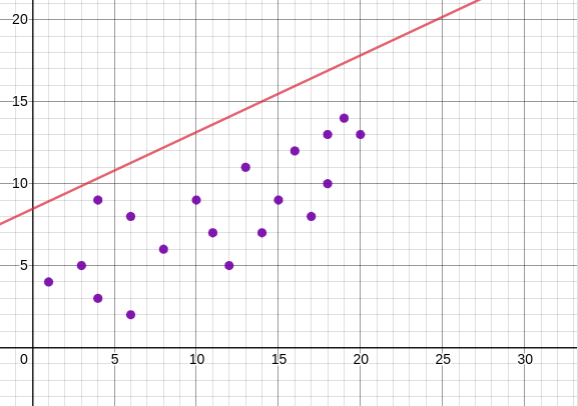# Linear Algebra - Vector Spaces Quiz 6 (Least Squares)

In the least squares problem we find that

$A^T Ax = A^T b$

and

$c_1 = \frac{\sum y_i}{n} , c_2 = \frac{\sum x_i y_i }{\sum x_i^2}$

where $y = c_1 + c_2 x$

I'm assuming this linear equation represents some kind of fitted line for the data. However, a previous step in the quiz involved setting all the $x_i$ coordinates to $x_i - \bar{x}$ to simplify finding $c_1$ and $c_2$.

So I went ahead and tried this method on a simple set I made up of 20 pieces of data of coordinates $(x,y)$:

$\{ (1,4), (3,5) , (4,3), (4,9), (6,2), (6,8), (8,6), (10,9), (11,7), (12, 5), (13,11), (14,7), (15,9), (16,12), (17,8), (18,10), (18,13), (19,14), (20,13), (20,14)\}$

A little spread out but I expect basically a positive linear relationship with y as a function of x.

Now I find with my calculator stat functions that $\bar{x} = 11.75$, so I set all $x_i$ to $x_i - 11.75$:

$X = \{ -10.75, -8.75, -7.75, -7.75, -5.75, -3.75, -1.75, -0.75, 0.25, 1.25, 2.25, 3.25, 4.25, 5.25, 6.25, 6.25, 7.25, 8.25, 8.25 \}$

My calculations from here:

$c_1 = \frac{\sum y_i}{n} = \frac{169}{20} = 8.45$ $c_2 = \frac{\sum x_i y_i}{\sum x_i^2} = \frac{340.25}{725.75} \approx 0.46883$

I wind up with a line equation that looks like

$y = 0.46883x + 8.45$

If I try and plot this, the line sits way outside any of my data.Did I miss some crucial step? Do the two constants change in subtracting the mean of X to begin with? Or am I misunderstanding the meaning of $c_1$ and $c_2$? As far as I can tell the quiz doesn't seem to suggest one way or another.Note by Jeff Folster
11 months, 3 weeks ago

This discussion board is a place to discuss our Daily Challenges and the math and science related to those challenges. Explanations are more than just a solution — they should explain the steps and thinking strategies that you used to obtain the solution. Comments should further the discussion of math and science.

When posting on Brilliant:

• Use the emojis to react to an explanation, whether you're congratulating a job well done , or just really confused .
• Ask specific questions about the challenge or the steps in somebody's explanation. Well-posed questions can add a lot to the discussion, but posting "I don't understand!" doesn't help anyone.
• Try to contribute something new to the discussion, whether it is an extension, generalization or other idea related to the challenge.

MarkdownAppears as
*italics* or _italics_ italics
**bold** or __bold__ bold
- bulleted- list
• bulleted
• list
1. numbered2. list
1. numbered
2. list
Note: you must add a full line of space before and after lists for them to show up correctly
paragraph 1paragraph 2

paragraph 1

paragraph 2

[example link](https://brilliant.org)example link
> This is a quote
This is a quote
    # I indented these lines
# 4 spaces, and now they show
# up as a code block.

print "hello world"
# I indented these lines
# 4 spaces, and now they show
# up as a code block.

print "hello world"
MathAppears as
Remember to wrap math in $$ ... $$ or $ ... $ to ensure proper formatting.
2 \times 3 $2 \times 3$
2^{34} $2^{34}$
a_{i-1} $a_{i-1}$
\frac{2}{3} $\frac{2}{3}$
\sqrt{2} $\sqrt{2}$
\sum_{i=1}^3 $\sum_{i=1}^3$
\sin \theta $\sin \theta$
\boxed{123} $\boxed{123}$